# Basic Oscilloscope Operation

## AC Electric Circuits

• #### Question 1

An oscilloscope is a very useful piece of electronic test equipment. Most everyone has seen an oscilloscope in use, in the form of a heart-rate monitor (electrocardiogram, or EKG) of the type seen in doctor’s offices and hospitals.

When monitoring heart beats, what do the two axes (horizontal and vertical) of the oscilloscope screen represent?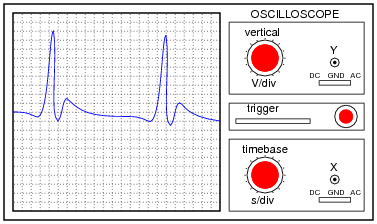In general electronics use, when measuring AC voltage signals, what do the two axes (horizontal and vertical) of the oscilloscope screen represent?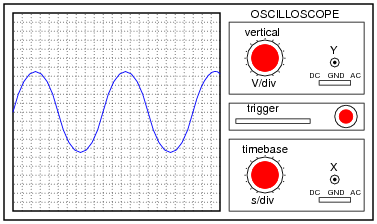• #### Question 2

The core of an analog oscilloscope is a special type of vacuum tube known as a Cathode Ray Tube, or CRT. While similar in function to the CRT used in televisions, oscilloscope display tubes are specially built for the purpose of serving an a measuring instrument.

Explain how a CRT functions. What goes on inside the tube to produce waveform displays on the screen?

• #### Question 3

When the vertical (“Y”) axis of an oscilloscope is shorted, the result should be a straight line in the middle of the screen: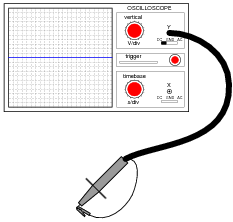Determine the DC polarity of the voltage source, based on this illustration: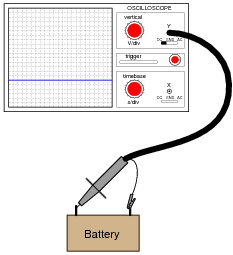• #### Question 4

An oscilloscope is connected to a battery of unknown voltage. The result is a straight line on the display: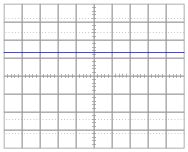Assuming the oscilloscope display has been properly “zeroed” and the vertical sensitivity is set to 5 volts per division, determine the voltage of the battery.

• #### Question 5

A technician prepares to use an oscilloscope to display an AC voltage signal. After turning the oscilloscope on and connecting the Y input probe to the signal source test points, this display appears: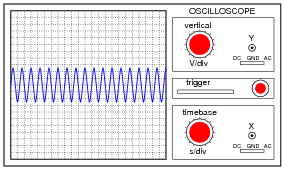What display control(s) need to be adjusted on the oscilloscope in order to show fewer cycles of this signal on the screen, with a greater height (amplitude)?

• #### Question 6

A technician prepares to use an oscilloscope to display an AC voltage signal. After turning the oscilloscope on and connecting the Y input probe to the signal source test points, this display appears: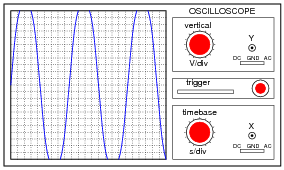What display control(s) need to be adjusted on the oscilloscope in order to show a normal-looking wave on the screen?

• #### Question 7

A technician prepares to use an oscilloscope to display an AC voltage signal. After turning the oscilloscope on and connecting the Y input probe to the signal source test points, this display appears: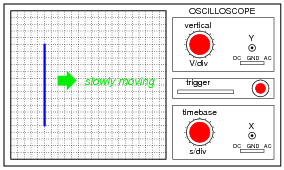What appears on the oscilloscope screen is a vertical line that moves slowly from left to right. What display control(s) need to be adjusted on the oscilloscope in order to show a normal-looking wave on the screen?

• #### Question 8

A technician prepares to use an oscilloscope to display an AC voltage signal. After turning the oscilloscope on and connecting the Y input probe to the signal source test points, this display appears: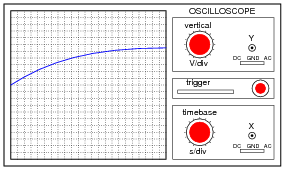What display control(s) need to be adjusted on the oscilloscope in order to show a normal-looking wave on the screen?

• #### Question 9

Determine the frequency of this waveform, as displayed by an oscilloscope with a vertical sensitivity of 2 volts per division and a timebase of 0.5 milliseconds per division: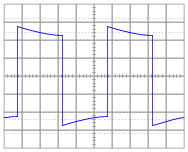• #### Question 10

Assuming the vertical sensitivity control is set to 2 volts per division, and the timebase control is set to 10 μs per division, calculate the amplitude of this “sawtooth” wave (in volts peak and volts peak-to-peak) as well as its frequency.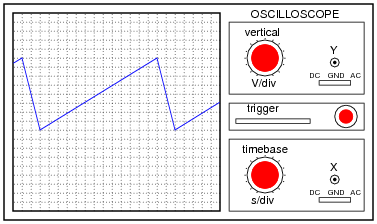• #### Question 11

Most oscilloscopes can only directly measure voltage, not current. One way to measure AC current with an oscilloscope is to measure the voltage dropped across a shunt resistor. Since the voltage dropped across a resistor is proportional to the current through that resistor, whatever wave-shape the current is will be translated into a voltage drop with the exact same wave-shape.

However, one must be very careful when connecting an oscilloscope to any part of a grounded system, as many electric power systems are. Note what happens here when a technician attempts to connect the oscilloscope across a shunt resistor located on the “hot” side of a grounded 120 VAC motor circuit: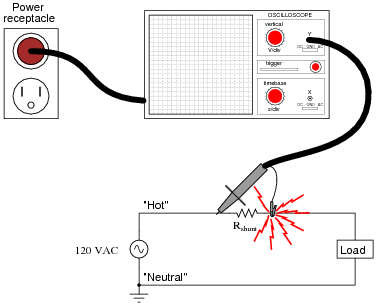Here, the reference lead of the oscilloscope (the small alligator clip, not the sharp-tipped probe) creates a short-circuit in the power system. Explain why this happens.

• #### Question 12

Most oscilloscopes have at least two vertical inputs, used to display more than one waveform simultaneously: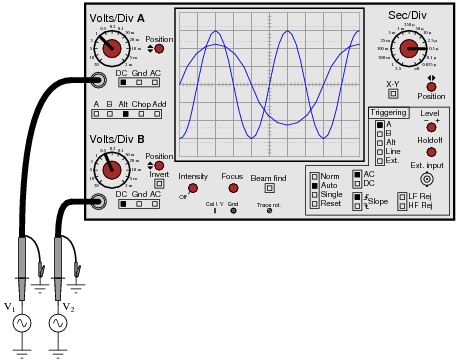While this feature is extremely useful, one must be careful in connecting two sources of AC voltage to an oscilloscope. Since the “reference” or “ground” clips of each probe are electrically common with the oscilloscope’s metal chassis, they are electrically common with each other as well.

Explain what sort of problem would be caused by connecting a dual-trace oscilloscope to a circuit in the following manner: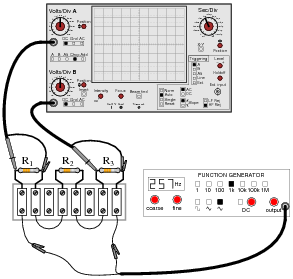• #### Question 13

Assuming the vertical sensitivity control is set to 0.5 volts per division, and the timebase control is set to 2.5 ms per division, calculate the amplitude of this sine wave (in volts peak, volts peak-to-peak, and volts RMS) as well as its frequency.• #### Question 14

Something is wrong with this circuit. Based on the oscilloscope’s display, determine whether the battery or the function generator is faulty: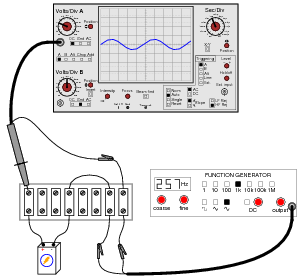• #### Question 15

Something is wrong with this circuit. Based on the oscilloscope’s display, determine whether the battery or the function generator is faulty: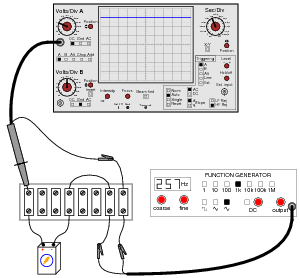• #### Question 16

Shunt resistors are low-value, precision resistors used as current-measuring elements in high-current circuits. The idea is to measure the voltage dropped across this precision resistance and use Ohm’s Law (I = V/R) to infer the amount of current in the circuit:Since the schematic shows a shunt resistor being used to measure current in an AC circuit, it would be equally appropriate to use an oscilloscope instead of a voltmeter to measure the voltage drop produced by the shunt. However, we must be careful in connecting the oscilloscope to the shunt because of the inherent ground reference of the oscilloscope’s metal case and probe assembly.

Explain why connecting an oscilloscope to the shunt as shown in this second diagram would be a bad idea: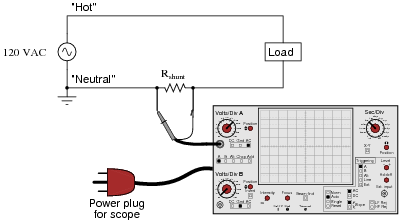• #### Question 17

An oscilloscope is connected to a battery of unknown voltage. The result is a straight line on the display: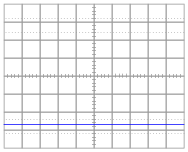Assuming the oscilloscope display has been properly “zeroed” and the vertical sensitivity is set to 2 volts per division, determine the voltage of the battery.

• #### Question 18

One of the more complicated controls to master on an oscilloscope, but also one of the most useful, is the triggering control. Without proper “triggering,” a waveform will scroll horizontally across the screen rather than staying “locked” in place.

Describe how the triggering control is able to “lock” an AC waveform on the screen so that it appears stable to the human eye. What, exactly, is the triggering function doing that makes an AC waveform appear to stand still?

• #### Question 19

If an oscilloscope is connected to a series combination of AC and DC voltage sources, what is displayed on the oscilloscope screen depends on where the “coupling” control is set.

With the coupling control set to “DC”, the waveform displayed will be elevated above (or depressed below) the “zero” line: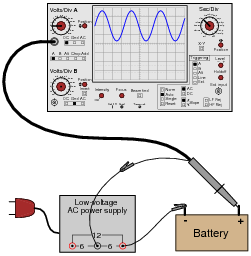Setting the coupling control to ÄC”, however, results in the waveform automatically centering itself on the screen, about the zero line.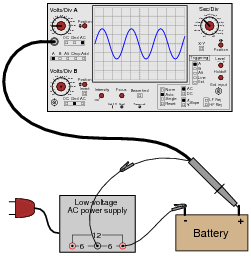Based on these observations, explain what the “DC” and ÄC” settings on the coupling control actually mean.

• #### Question 20

Explain what happens inside an oscilloscope when the “coupling” switch is moved from the “DC” position to the ÄC” position.

• #### Question 21

Suppose a technician measures the voltage output by an AC-DC power supply circuit: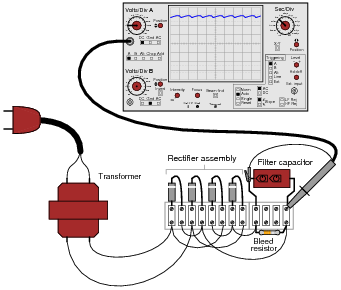The waveform shown by the oscilloscope is mostly DC, with just a little bit of AC “ripple” voltage appearing as a ripple pattern on what would otherwise be a straight, horizontal line. This is quite normal for the output of an AC-DC power supply.

Suppose we wished to take a closer view of this “ripple” voltage. We want to make the ripples more pronounced on the screen, so that we may better discern their shape. Unfortunately, though, when we decrease the number of volts per division on the “vertical” control knob to magnify the vertical amplification of the oscilloscope, the pattern completely disappears from the screen!

Explain what the problem is, and how we might correct it so as to be able to magnify the ripple voltage waveform without having it disappear off the oscilloscope screen.

• #### Question 22

A student just learning to use oscilloscopes connects one directly to the output of a signal generator, with these results: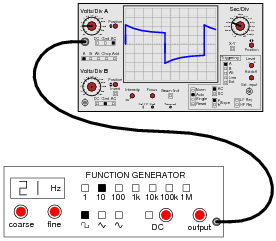As you can see, the function generator is configured to output a square wave, but the oscilloscope does not register a square wave. Perplexed, the student takes the function generator to a different oscilloscope. At the second oscilloscope, the student sees a proper square wave on the screen: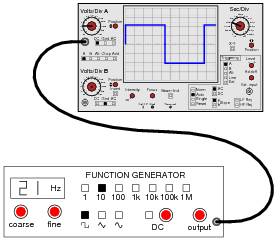It is then that the student realizes the first oscilloscope has its “coupling” control set to AC, while the second oscilloscope was set to DC. Now the student is really confused! The signal is obviously AC, as it oscillates above and below the centerline of the screen, but yet the “DC” setting appears to give the most accurate results: a true-to-form square wave.

How would you explain what is happening to this student, and also describe the appropriate uses of the ÄC” and “DC” coupling settings so he or she knows better how to use it in the future?

• #### Question 23

There are times when you need to use an oscilloscope to measure a differential voltage that also has a significant common-mode voltage: an application where you cannot connect the oscilloscope’s ground lead to either point of contact. One application is measuring the voltage pulses on an RS-485 digital communications network, where neither conductor in the two-wire cable is at ground potential, and where connecting either wire to ground (via the oscilloscope’s ground clip) may cause problems: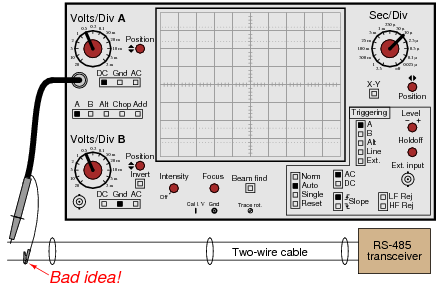One solution to this problem is to use both probes of a dual-trace oscilloscope, and set it up for differential measurement. In this mode, only one waveform will be shown on the screen, even though two probes are being used. No ground clips need be connected to the circuit under test, and the waveform shown will be indicative of the voltage between the two probe tips.

Describe how a typical oscilloscope may be set up to perform differential voltage measurement. Be sure to include descriptions of all knob and button settings (with reference to the oscilloscope shown in this question):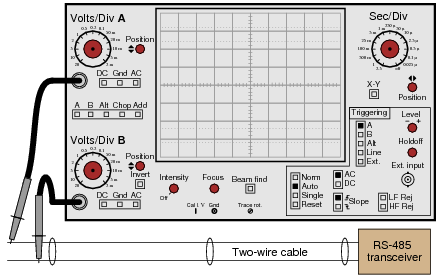• #### Question 24

A very common accessory for oscilloscopes is a ×10 probe, which effectively acts as a 10:1 voltage divider for any measured signals. Thus, an oscilloscope showing a waveform with a peak-to-peak amplitude of 4 divisions, with a vertical sensitivity setting of 1 volt per division, using a ×10 probe, would actually be measuring a signal of 40 volts peak-peak: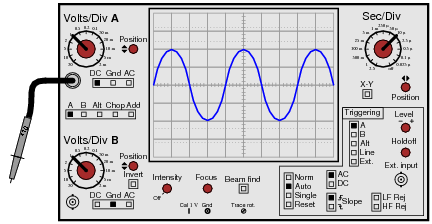Obviously, one use for a ×10 probe is measuring voltages beyond the normal range of an oscilloscope. However, there is another application that is less obvious, and it regards the input impedance of the oscilloscope. A ×10 probe gives the oscilloscope 10 times more input impedance (as seen from the probe tip to ground). Typically this means an input impedance of 10 MΩ (with the ×10 probe) rather than 1 MΩ (with a normal 1:1 probe). Identify an application where this feature could be useful.

• #### Question 25

Explain what an active probe is for an oscilloscope, and why they are useful for some measurement applications.

### Related Content

Published under the terms and conditions of the Creative Commons Attribution License•terezkaterka June 04, 2020